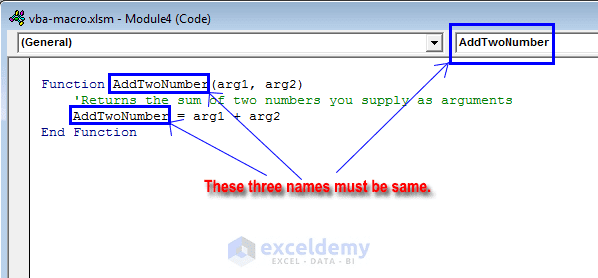Disclosure: This post may contain affiliate links, meaning when you click the links and make a purchase, we receive a commission.

# 5 Differences Between Subroutine & Function in Excel VBA

There are lots of similarities between a custom Function/User-Defined Function procedure and the Sub-procedure/Subroutine. However, Function procedures have some important differences with subroutine procedures. In this article, we will see the difference between subroutine and function in Excel VBA.

The most important difference is that a function returns a value (a number or a text string). The value of the function procedure is stored in a variable; a variable whose name is same as function’s name. The subroutine performs some set of task and does not return a value like functions.

## Excel VBA User-Defined Function

See the following example. AddTwoNumber is the function name. This function will return the sum of two numbers passed as arguments (arg1 and arg2). The sum is stored in a variable named AddTwoNumber same as the function name.

```Function AddTwoNumber(arg1, arg2)
'Returns the sum of two numbers you supply as arguments
End Function```### To Create a Custom Function, Follow These Steps:

1. Activate the VB Editor (press Alt+F11).
2. Select the workbook in the Project window.
3. Choose Insert ➪ Module to insert a VBA module. You can also use an existing code module. The code module must be a standard VBA module.
4. Enter the keyword Function followed by the function’s name. The function name must be unique for that workbook. Enter a list of the arguments (if any) in parentheses. If the function doesn’t use an argument, the VB Editor adds a set of empty parentheses.
5. This part is important. Insert the VBA code that performs your intended objective. The value what you want to return from this function will be stored in a variable; a variable whose name is the same as function’s name.
6. End the function with an End Function statement.

## Excel VBA Subroutine

In the following example, you will see how the subroutine in Excel VBA works. Here the Sub starts the body of the subroutine. The subroutine name is square_root. In the body of the subroutine, we perform a task which in cell A2. The task is performing the square root in cell A2. It means, if the cell contains any number, the Excel VBA will perform the square root of that cell. The End Sub ends the body of the subroutine.

### To Create a Subroutine, Follow These Steps:

1. Activate the VB Editor (press Alt+F11).
2. Select the workbook in the Project window.
3. Choose Insert ➪ Module to insert a VBA module. You can also use an existing code module. The code module must be a standard VBA module.
4. Enter the keyword SUB followed by the Subroutine’s name.
5. Insert the VBA code that you want to perform.
6. End the Subroutine with an End Sub statement.

## Differences Between Subroutine & Function in Excel VBA

After performing the subroutine and functions separately we can conclude the differences in the table below.

FunctionsSubroutines
1) Return a value1) Performs a set of tasks but doesn’t return a value
2) Functions are called by using a variable2) Can be recalled from anywhere within the program in multiple types after the declaration
3) Can be used as formulas in the spreadsheets3) Cannot be used directly in the spreadsheets as formulas
4) As functions can be used as a formula in the spreadsheets. You can perform it several times after running the code.4) To find the result of the Excel VBA subroutine you have to insert a value in the desired cell first.
5) Syntax:

Function Function_Name()

//Set of codes

End Function

5) Syntax:

Sub Sub_Name ()

//Set of codes

End Sub